﻿ Excel SUMIF函数的典型用法总结 - 自由资讯

# Excel SUMIF函数的典型用法总结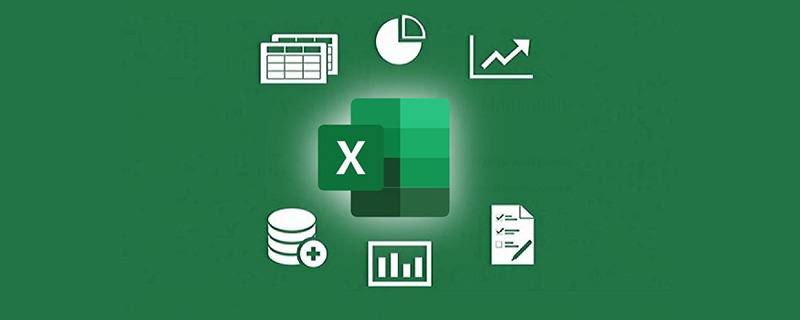SUMIF函数用于对区域中符合条件的值求和。她只有3个参数：

=SUMIF(条件区域,指定的条件,需要求和的区域)

SUMIF函数真的这么简单吗？今天咱们就一起领略一下这个函数的典型用法。

## 1、返回如花的总额

=SUMIF(B:B,”如花”,C:C)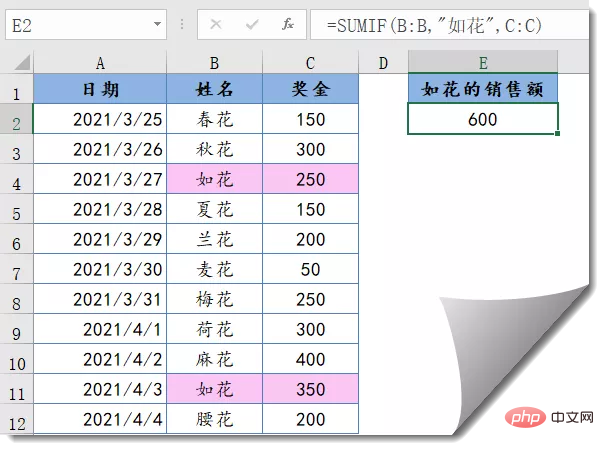## 2、返回除了如花之外的总额

=SUMIF(C:C,”>900″)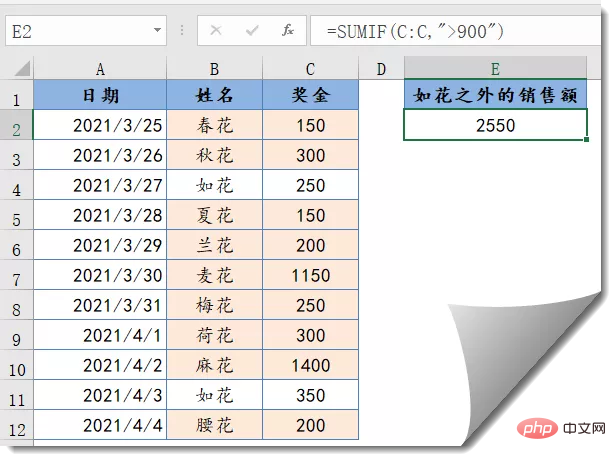## 3、大于900的总额

=SUMIF(C:C,”>900″)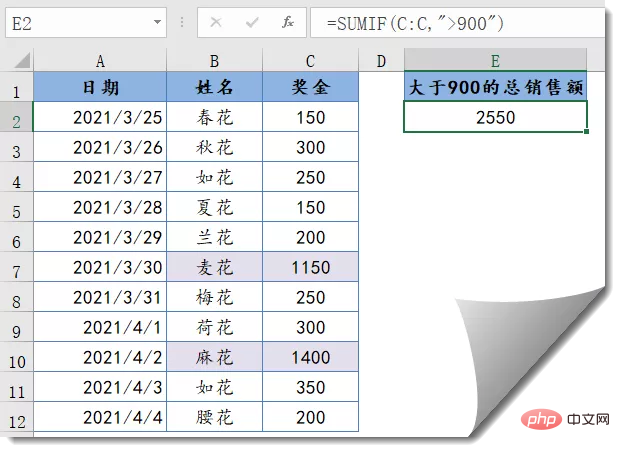## 4、低于平均值的总额

=SUMIF(C:C,”<“&AVERAGE(C:C))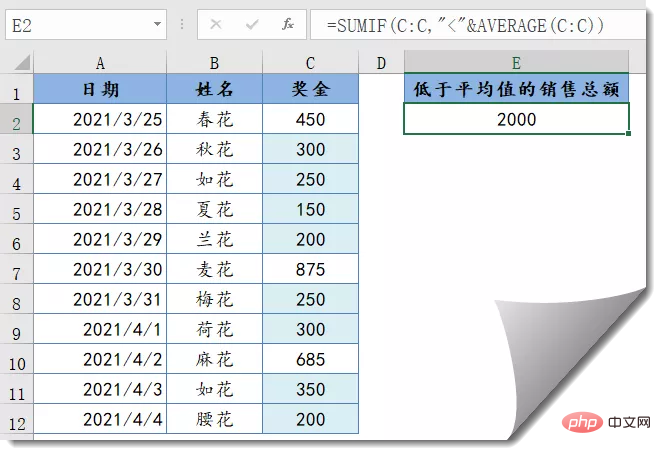## 5、返回姓名中包含“花”的总金额

=SUMIF(B:B,”*花*”,C:C)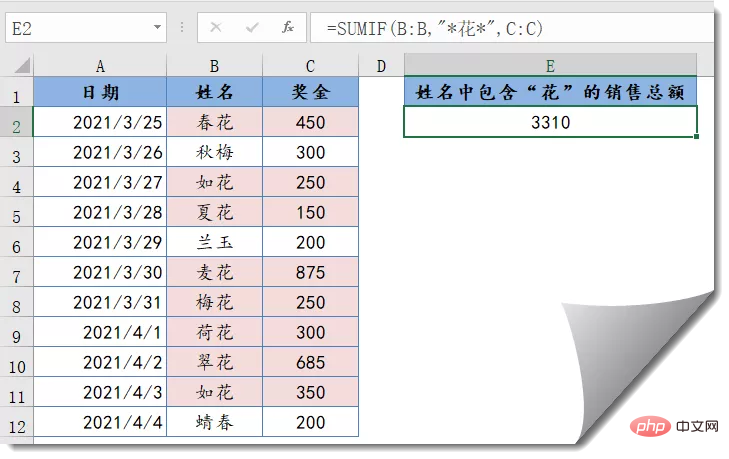## 6、返回当前日期之前的金额

=SUMIF(A:A,”<“&TODAY(),C:C)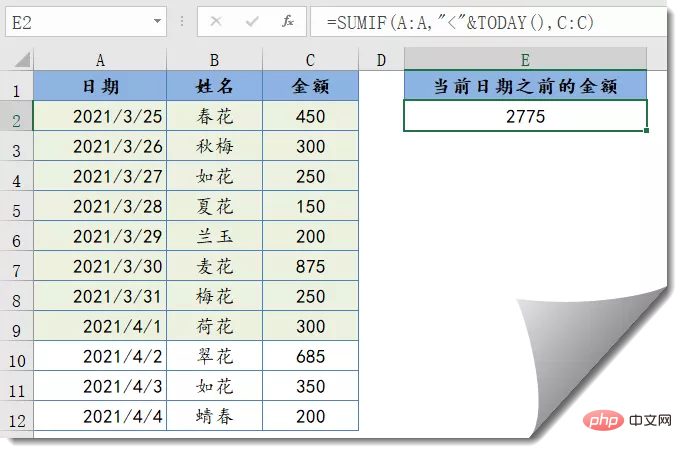## 7、忽略错误值求和

=SUMIF(C:C,”<9e307″)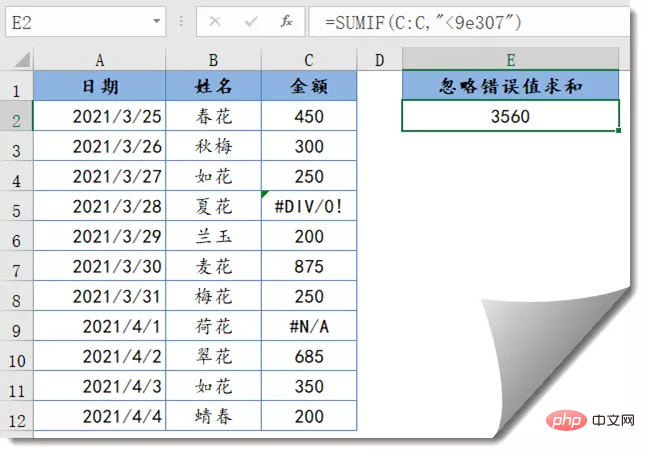## 8、返回如花和秋花的总额

=SUM(SUMIF(B:B,{“如花”,”秋花”},C:C))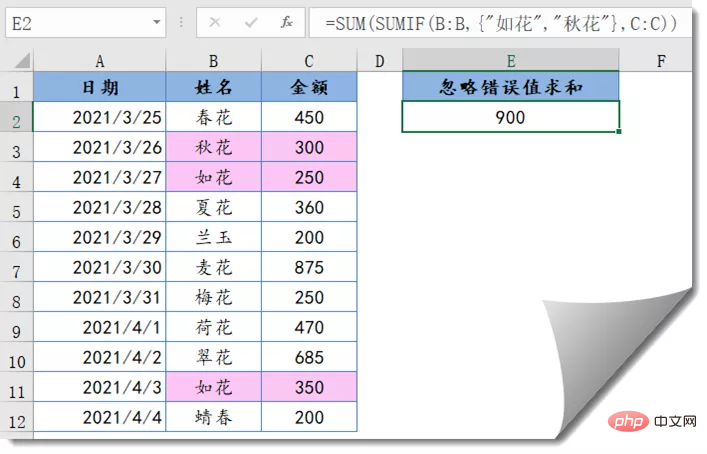## 9、错列求和

=SUMIF(B:H,K3,C:I)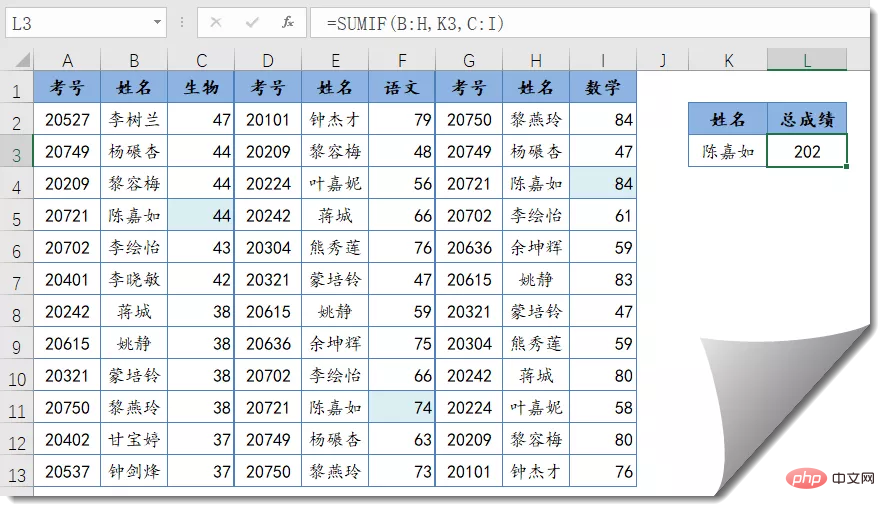﻿